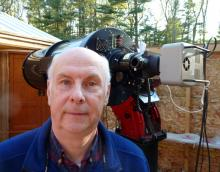# BSM_NH2 Transformation Coefficients

8 posts / 0 new
MZKBSM_NH2 Transformation Coefficients

BSM_NH2 Transformation Coefficients

Attached are the BSM_NH2 Transformation Coefficients generated in December 2018. Thay are attached as a .ini file that can be opened with a text editor. This .ini file, after deleting the _.txt extension, can be loaded directly into Transform Applier.

Note that the BSM_NH2 filter wheel contains a Sloan SR filter (not a Rc filter) and thus the related coefficients transform magnitudes to the Rc standard magnitude.

The coeffs, which follow, have been added to the VPhot telescope setup.

Tbv= 1.098
Tb_bv= 0.030
Tv_bv= -0.045
Tvr= 1.294
Tv_vr= -0.087
Tr_vr= -0.257
Tri= 0.825
Tr_ri= -0.308
Ti_ri= -0.047
Tvi= 1.000
Tv_vi= -0.040
Ti_vi= -0.026
Tr_vi= -0.146NH2 Transform Coeff 181217.ini_.txt
sppBSM-NH2

Ken,

What camera does BSM-NH2 use?

Phil

HQABSM_NH2 camera

Hi Phil,

BSM_NH2 is using the ZWO ASI-183mm-cool-pro camera.  This is a 12-bit CMOS system, but used in a 2x2 binning mode which essentially gives 14-bit precision.  ZWO's largest filter wheel has 8 positions, so we opted to remove the Rc filter and use SR (Sloan r) when R-band photometry was needed.  That is why Ken shows transformation coefficients using SR that are larger than what you normally see for the typical Astrodon Rc filter.

The full BSM_NH2 filter set is B,V,Ic,u,g,r,i,z_s.  It lost the Rc filter and the SA200 diffraction grating from the original BSM_NH filter set.  It has a wide spectral range coverage from the Sloan set, part of the ongoing testing of the capabilities of these CMOS cameras.  So far, I'm pleased with what I see.

We're waiting for MaximDL to implement a real-time stacking option, at which point we'll be able to go far brighter than the original BSM, and also be able to stack 4 images/filter before saving to obtain 16-bit precision on other fields.

Arne

sppBSM_NH2 camera

Hi Arne,

Thanks for the update on what you have been doing with the CMOS camera.   Do you have a guestimate of how bright you'll be able to observe when MaximDL can stack in real time?

Phil

HQAHi Phil,

Hi Phil,

If things work as they are described, we should be able to do everything, including Sirius.  The big problem is scintillation.  For Sirius, the exposures are about 0.01sec, and you need to coadd 1000 such exposures to reduce scintillation.  For obvious reasons, you want to do this stacking in real time and just write the result to disk.  If we can accomplish this, we won't even need to defocus.

Arne

sppobserving Sirius with CMOS camera

That would be quite a feat.   What would you use for comparison stars?  Would you have to use all-sky photometry?

Phil

HQAsirius

Hi Phil,

Yes, Sirius is an exception.  In order to image it, you have to seriously subframe, and so there would be little chance for a nearby star to use as a comparison.  So for it, you'd have to work in the PEP mode, using a comp star a degree or more away.

For fainter stars, say mag 0 like Vega, you can image full frame, and so can use comp stars as faint as mag 6 or 7 since you are stacking ~20 seconds worth of exposures.

Arne

MZKBSM_NH2 Transformation Coefficients 190510

BSM_NH2 Transformation Coefficients

Attached are the BSM_NH2 Transformation Coefficients generated in May 2019. Thay are attached as a .ini file that can be opened with a text editor. This .ini file, after deleting the _.txt extension, can be loaded directly into Transform Applier.

Note that the BSM_NH2 filter wheel contains a Sloan SR filter (not a Rc filter) and thus the related coefficients transform magnitudes to the Rc standard magnitude.

The coeffs, which follow, have been added to the VPhot telescope setup.

[Setup]
description= TG - Version TG_V7.3 beta 5, Telescope= NH2, Time created (UT) = 2019_05_10_01:27:46
[Coefficients]
Tbv= 1.054
Tb_bv= -0.033
Tv_bv= -0.079
Tvr= 1.094
Tv_vr= -0.160
Tr_vr= -0.249
Tri= 0.877
Tr_ri= -0.307
Ti_ri= -0.143
Tvi= 0.985
Tv_vi= -0.091
Ti_vi= -0.062
Tr_vi= -0.135
[Error]
Tbv= 0.035
Tb_bv= 0.036
Tv_bv= 0.025
Tvr= 0.030
Tv_vr= 0.049
Tr_vr= 0.050
Tri= 0.030
Tr_ri= 0.051
Ti_ri= 0.051
Tvi= 0.019
Tv_vi= 0.027
Ti_vi= 0.023
Tr_vi= 0.022
[R Squared Values]
Tbv= 0.978
Tb_bv= 0.038
Tv_bv= 0.300
Tvr= 0.989
Tv_vr= 0.373
Tr_vr= 0.659
Tri= 0.981
Tr_ri= 0.688
Ti_ri= 0.235
Tvi= 0.993
Tv_vi= 0.392
Ti_vi= 0.231
Tr_vi= 0.669

KenNH2 Transform Coeff 190508.ini_.txt